• 图像形成–采样和量化
• 离散傅里叶变换
• 理解卷积

# 图像形成–采样和量化

% matplotlib inline # for inline image display inside notebookfrom PIL import Imagefrom skimage.io import imread, imshow, showimport scipy.fftpack as fpfrom scipy import ndimage, misc, signalfrom scipy.stats import signaltonoisefrom skimage import data, img_as_floatfrom skimage.color import rgb2grayfrom skimage.transform import ...


# 上采样

• 聚合，例如，其最近的一个或多个已知像素邻域值的平均值
• 使用像素邻域进行双线性或三次插值的插值

im = Image.open("../images/clock.jpg") # the original small ...


# 上采样和插值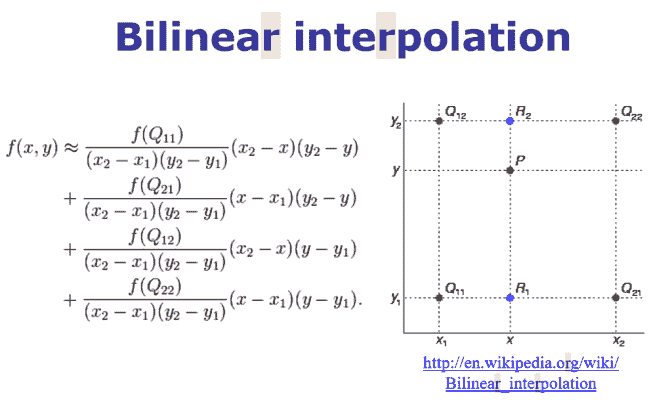im1 = im.resize((im.width*5, im.height*5), Image.BILINEAR) # up-sample with bi-linear interpolation
pylab.figure(figsize=(10,10)), pylab.imshow(im1), pylab.show()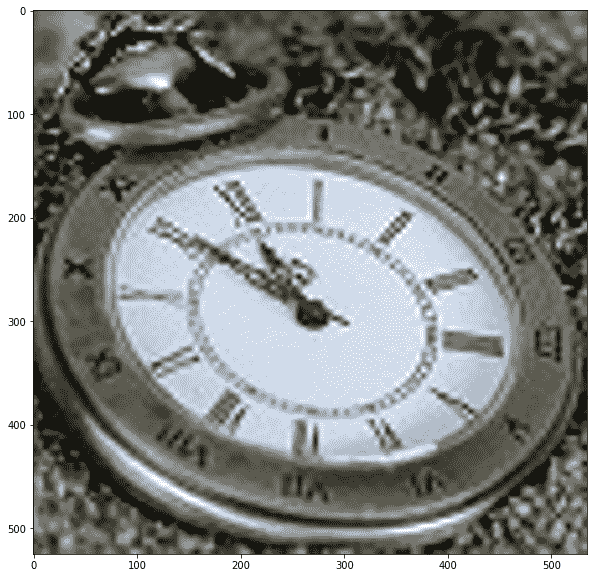im.resize((im.width*10, im.height*10), Image.BICUBIC).show()  # bi-cubic interpolation
pylab.figure(figsize=(10,10)), pylab.imshow(im1), pylab.show()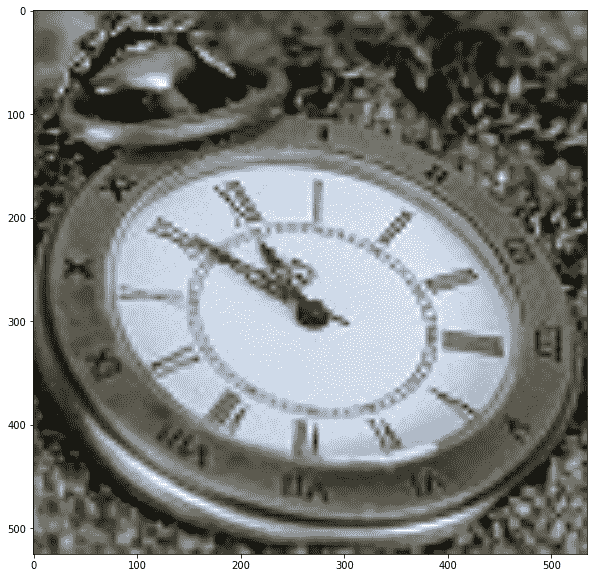# 下采样

• 以系统的方式从较大的图像中删除一些像素（例如，如果我们希望图像的大小为原始图像的四分之一，则每隔一行和一列删除一个像素）
• 计算新像素值作为原始图像中对应的多个像素的聚合值

im = Image.open("../images/tajmahal.jpg") ...


# 下采样和抗锯齿

im = im.resize((im.width//5, im.height//5), Image.ANTIALIAS)
pylab.figure(figsize=(15,10)), pylab.imshow(im), pylab.show()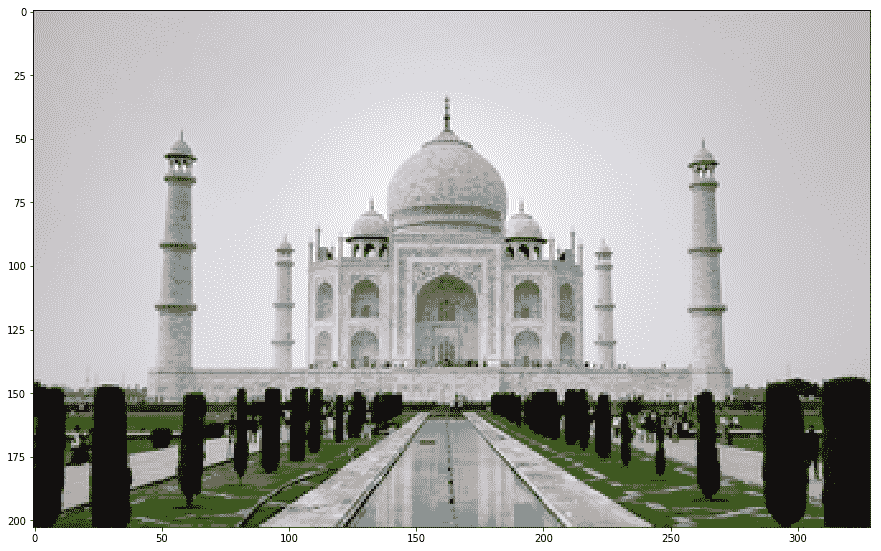im = imread('../images/umbc.png')
im1 = im.copy()
pylab.figure(figsize=(20,15))
for i in range(4):
pylab.subplot(2,2,i+1), pylab.imshow(im1, cmap='gray'), pylab.axis('off')
pylab.title('image size = ' + str(im1.shape) + 'x' + str(im1.shape))
im1 = rescale(im1, scale = 0.5, multichannel=True, anti_aliasing=False)
pylab.show()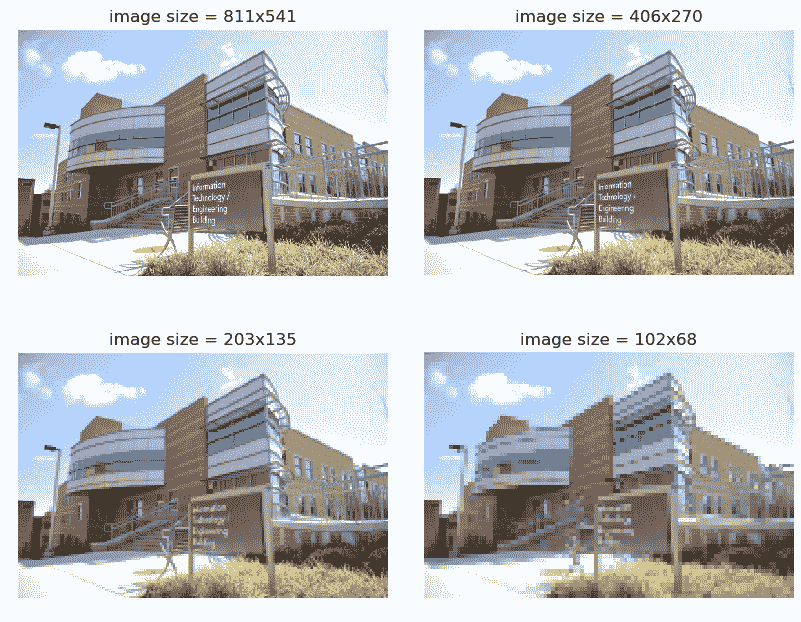im1 = rescale(im1, scale = 0.5, multichannel=True, anti_aliasing=True)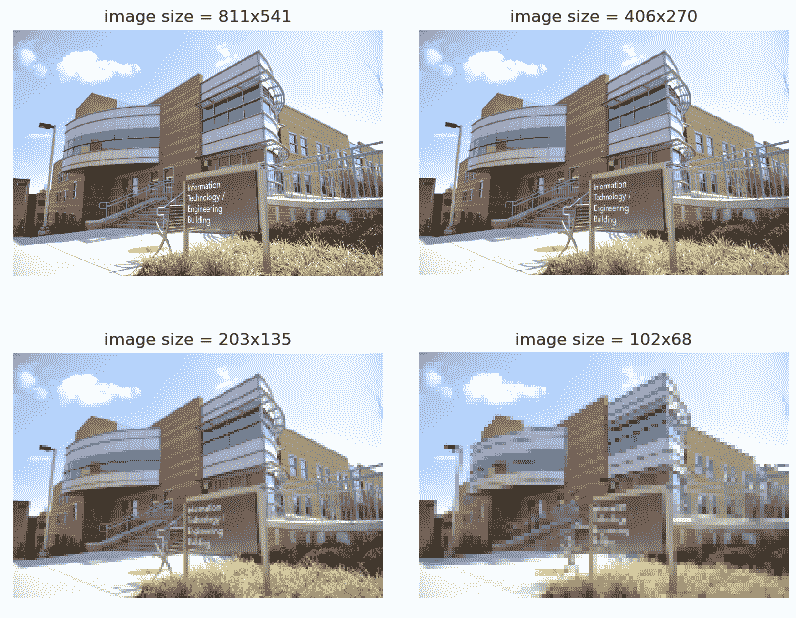# PIL 量子化

im = Image.open('../images/parrot.jpg')
pylab.figure(figsize=(20,30))
num_colors_list = [1 << n for n in range(8,0,-1)]
snr_list = []
i = 1
for num_colors in num_colors_list:
pylab.subplot(4,2,i), pylab.imshow(im1), pylab.axis('off')
snr_list.append(signaltonoise(im1, axis=None))
pylab.title('Image with # colors = ' + str(num_colors) + ' SNR = ' +
str(np.round(snr_list[i-1],3)), size=20)
i += 1
pylab.show()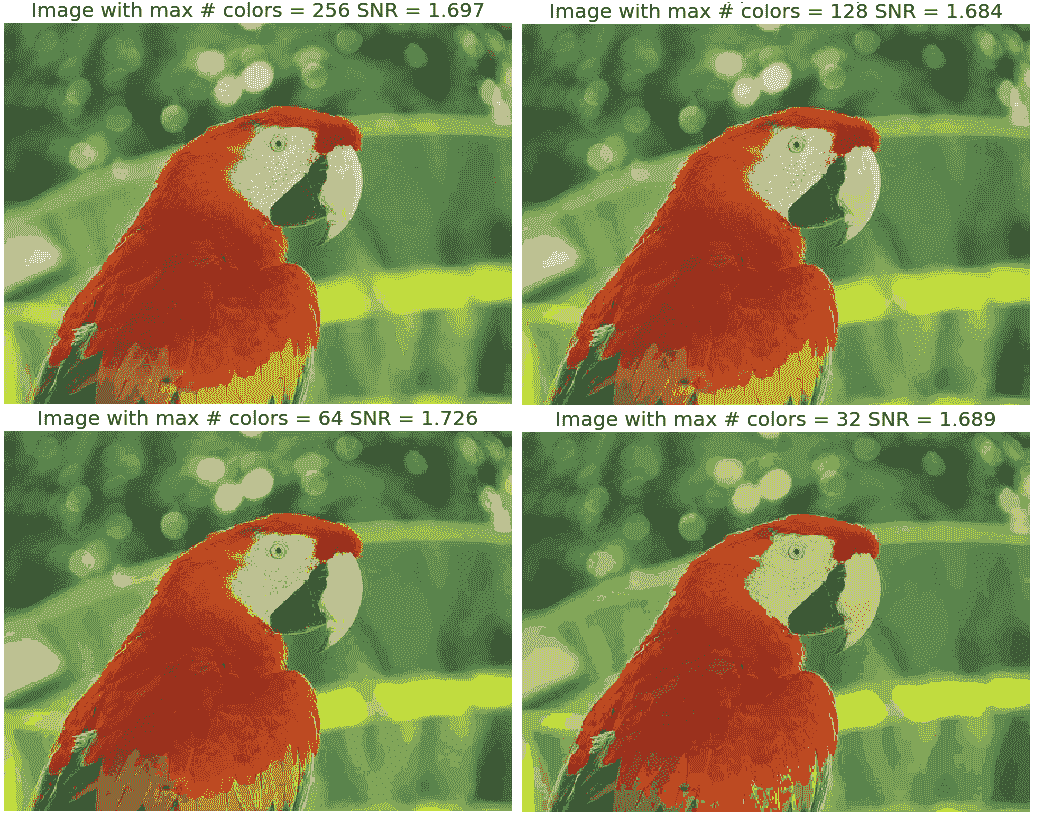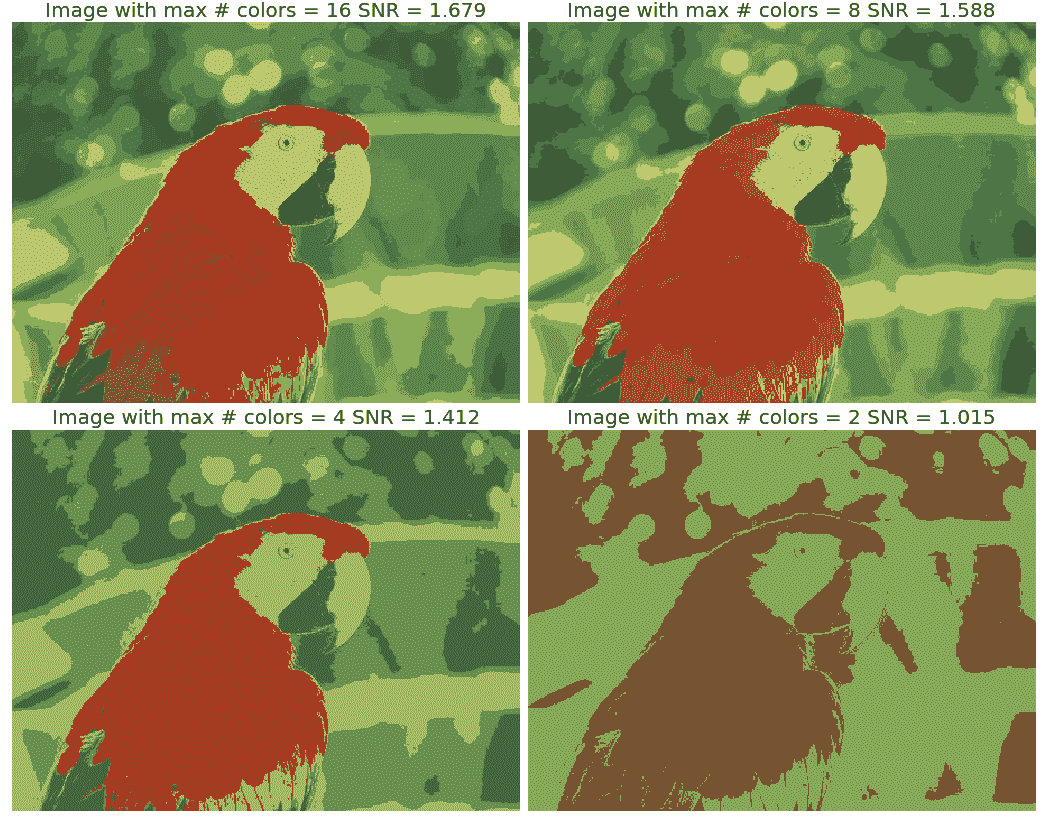pylab.plot(num_colors_list, snr_list, 'r.-')
pylab.xlabel('# colors in the image')
pylab.ylabel('SNR')
pylab.title('Change in SNR w.r.t. # colors')
pylab.xscale('log', basex=2)
pylab.gca().invert_xaxis()
pylab.show()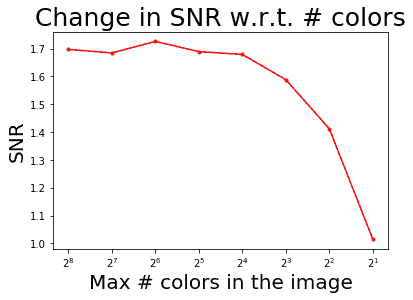# 计算 DFT 的快速傅里叶变换算法

*# 带有 scipy.fftpack 模块的 FFT

im = np.array(Image.open('../images/rhino.jpg').convert('L')) # we shall work with grayscale image
snr = signaltonoise(im, axis=None)
print('SNR for the original image = ' + str(snr))
# SNR for the original image = 2.023722773801701
# now call FFT and IFFT
freq = fp.fft2(im)
im1 = fp.ifft2(freq).real
snr = signaltonoise(im1, axis=None)
print('SNR for the image obtained after reconstruction = ' + str(snr))
# SNR for the image obtained after reconstruction = 2.0237227738013224
assert(np.allclose(im, im1)) # make sure the forward and inverse FFT are close to each other
pylab.figure(figsize=(20,10))
pylab.subplot(121), pylab.imshow(im, cmap='gray'), pylab.axis('off')
pylab.title('Original Image', size=20)
pylab.subplot(122), pylab.imshow(im1, cmap='gray'), pylab.axis('off')
pylab.title('Image obtained after reconstruction', size=20)
pylab.show()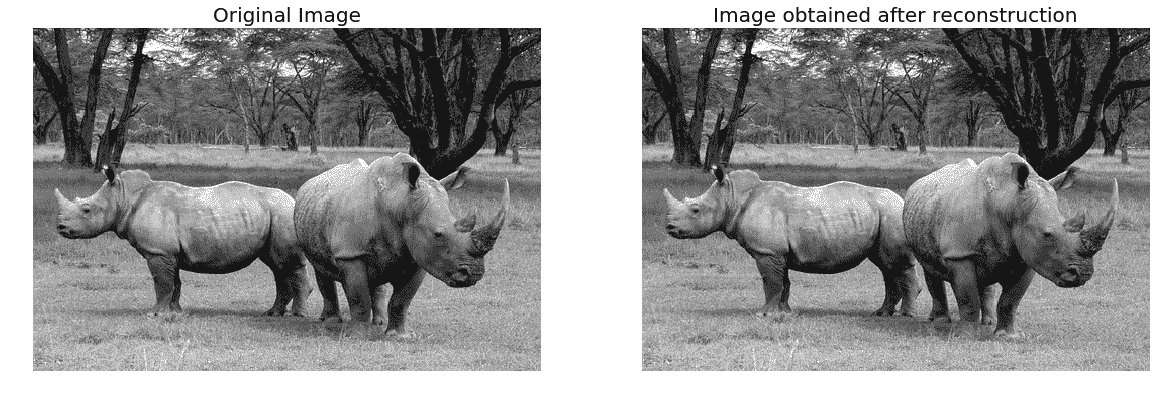# 绘制频谱图

DC 系数太大，无法看到其他系数值，这就是为什么我们需要通过显示变换的对数来拉伸变换值。此外，为了便于显示，变换系数被移位（使用fftshift()），使得 DC 分量位于中心。对创造犀牛图像的傅里叶光谱感到兴奋吗？编码如下：

# the quadrants are needed to be shifted around in order that the low spatial frequencies are in the center of the 2D fourier-transformed ...


# 计算 DFT 的幅度和相位

import numpy.fft as fpim1 = rgb2gray(imread('../images/house.png'))pylab.figure(figsize=(12,10))freq1 = fp.fft2(im1)im1_ = fp.ifft2(freq1).realpylab.subplot(2,2,1), pylab.imshow(im1, cmap='gray'), pylab.title('Original Image', size=20)pylab.subplot(2,2,2), pylab.imshow(20*np.log10( 0.01 + np.abs(fp.fftshift(freq1))), cmap='gray')pylab.title('FFT Spectrum Magnitude', size=20)pylab.subplot(2,2,3), pylab.imshow(np.angle(fp.fftshift(freq1)), cmap='gray')pylab.title('FFT ...


# 理解卷积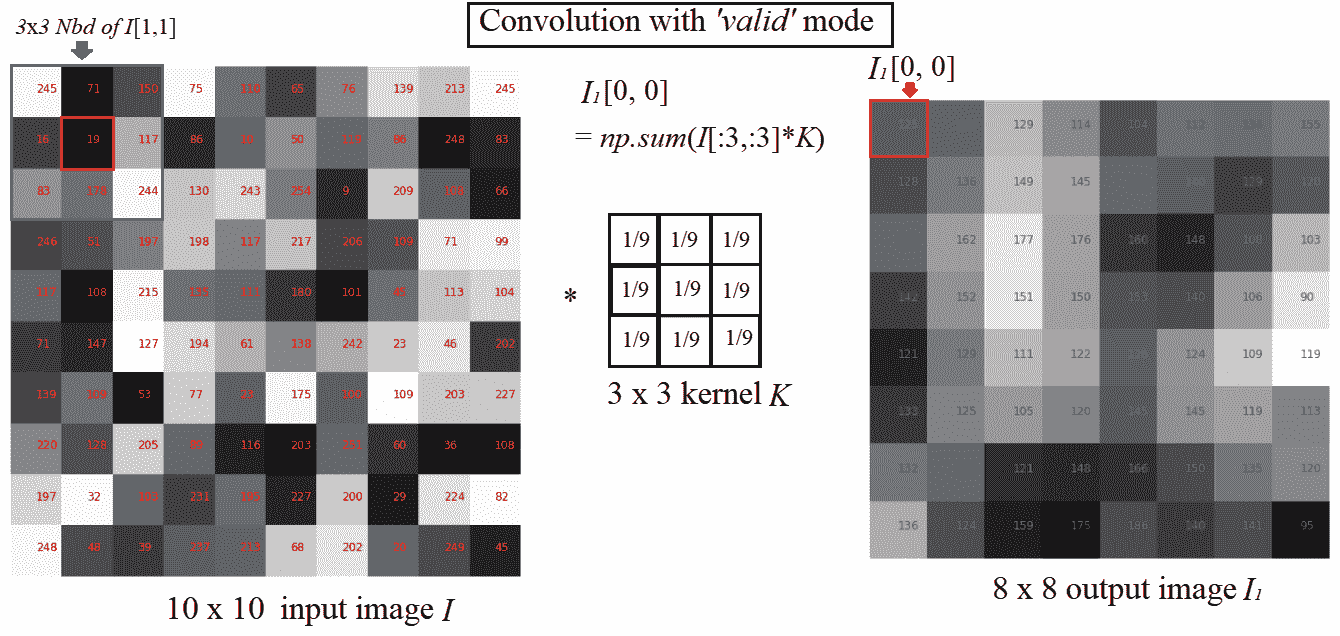# SciPy 信号卷积的卷积

SciPy 信号模块的convolve2d()功能可用于相关。我们将使用这个函数对具有内核的图像应用卷积。

# 对灰度图像应用卷积

im = rgb2gray(imread('../image s/cameraman.jpg')).astype(float)print(np.max(im))# 1.0print(im.shape)# (225, 225)blur_box_kernel = np.ones((3,3)) / 9edge_laplace_kernel = np.array([[0,1,0],[1,-4,1],[0,1,0]])im_blurred = signal.convolve2d(im, blur_box_kernel)im_edges = np.clip(signal.convolve2d(im, edge_laplace_kernel), 0, 1)fig, axes = pylab.subplots(ncols=3, sharex=True, sharey=True, figsize=(18, 6))axes.imshow(im, cmap=pylab.cm.gray)axes.set_title('Original Image', size=20)axes.imshow(im_blurred, cmap=pylab.cm.gray)axes.set_title('Box Blur', ...


# 卷积模式、焊盘值和边界条件

• mode='full'：默认模式，输出为输入的全离散线性卷积
• mode='valid'：忽略边缘像素，只计算所有相邻像素（不需要零填充的像素）。输出图像大小小于所有内核的输入图像大小（1 x 1 除外）
• mode='same'：输出图像与输入图像大小相同；它相对于'full'输出居中。

# 对彩色（RGB）图像应用卷积

im = misc.imread('../images/tajmahal.jpg')/255 # scale each pixel value in [0,1]print(np.max(im))print(im.shape)emboss_kernel = np.array([[-2,-1,0],[-1,1,1],[0,1,2]])edge_schar_kernel = np.array([[ -3-3j, 0-10j, +3 -3j], [-10+0j, 0+ 0j, +10+0j], [ -3+3j, 0+10j, +3 +3j]])im_embossed = np.ones(im.shape)im_edges = np.ones(im.shape)for i in range(3):    im_embossed[...,i] = np.clip(signal.convolve2d(im[...,i], emboss_kernel, mode='same', boundary="symm"),0,1)for i in range(3): ...


# 带 SciPy ndimage.COLVEL 的卷积

victoria_memorial.png映像与sharpen内核和emboss内核一起使用：

im = misc.imread('../images/victoria_memorial.png').astype(np.float) # read as float
print(np.max(im))
sharpen_kernel = np.array([0, -1, 0, -1, 5, -1, 0, -1, 0]).reshape((3, 3, 1))
emboss_kernel = np.array(np.array([[-2,-1,0],[-1,1,1],[0,1,2]])).reshape((3, 3, 1))
im_sharp = ndimage.convolve(im, sharpen_kernel, mode='nearest')
im_sharp = np.clip(im_sharp, 0, 255).astype(np.uint8) # clip (0 to 255) and convert to unsigned int
im_emboss = ndimage.convolve(im, emboss_kernel, mode='nearest')
im_emboss = np.clip(im_emboss, 0, 255).astype(np.uint8)
pylab.figure(figsize=(10,15))
pylab.subplot(311), pylab.imshow(im.astype(np.uint8)), pylab.axis('off')
pylab.title('Original Image', size=25)
pylab.subplot(312), pylab.imshow(im_sharp), pylab.axis('off')
pylab.title('Sharpened Image', size=25)
pylab.subplot(313), pylab.imshow(im_emboss), pylab.axis('off')
pylab.title('Embossed Image', size=25)
pylab.tight_layout()
pylab.show()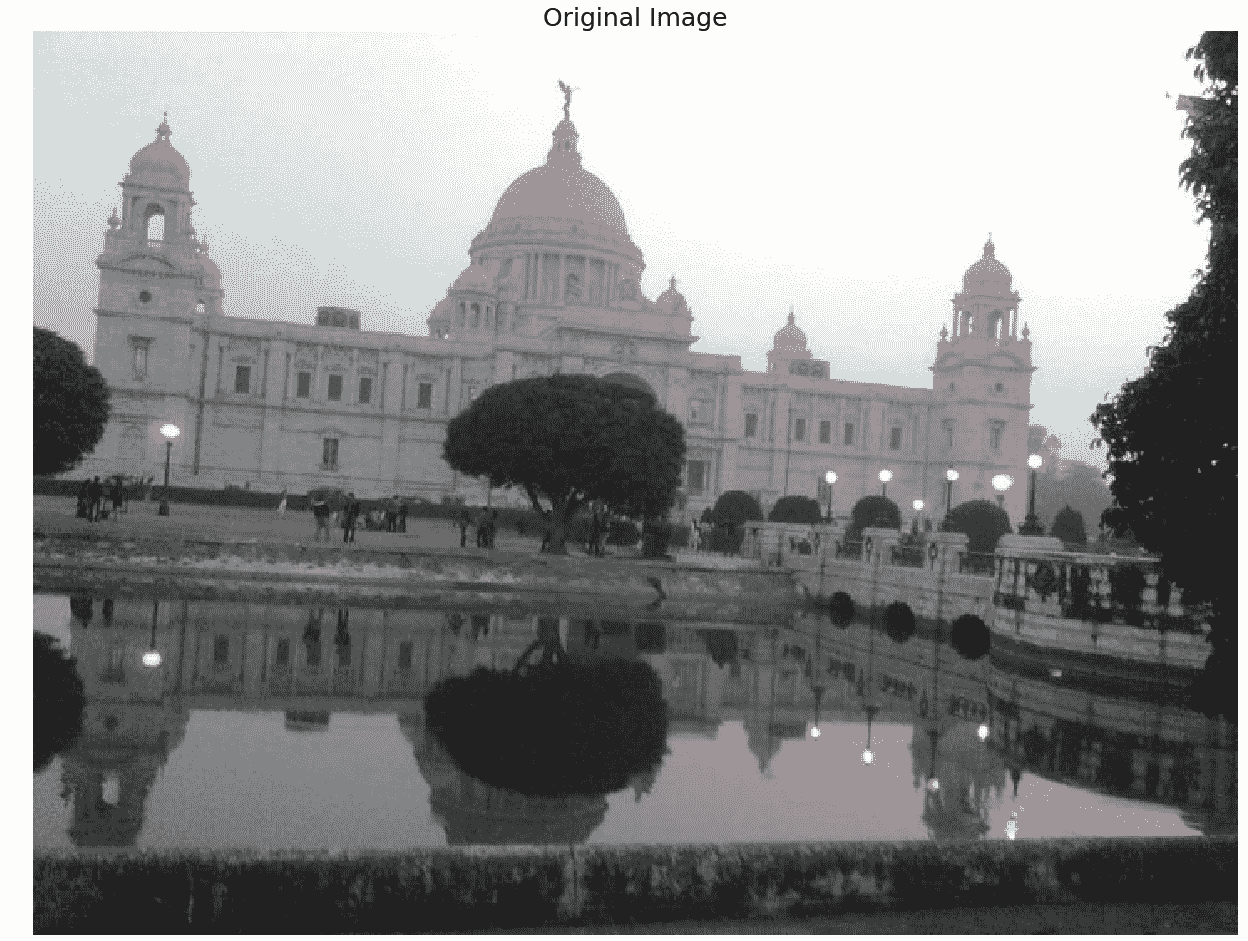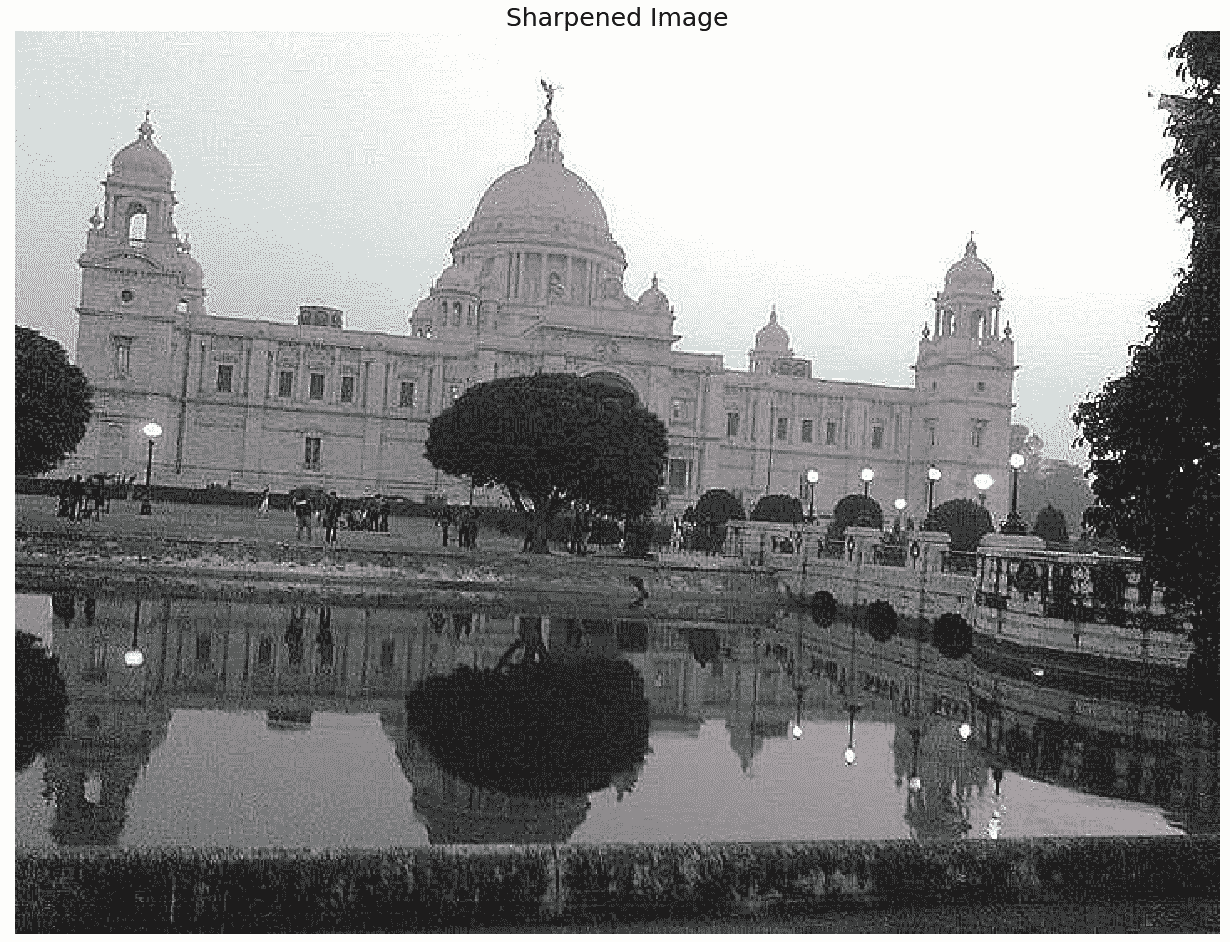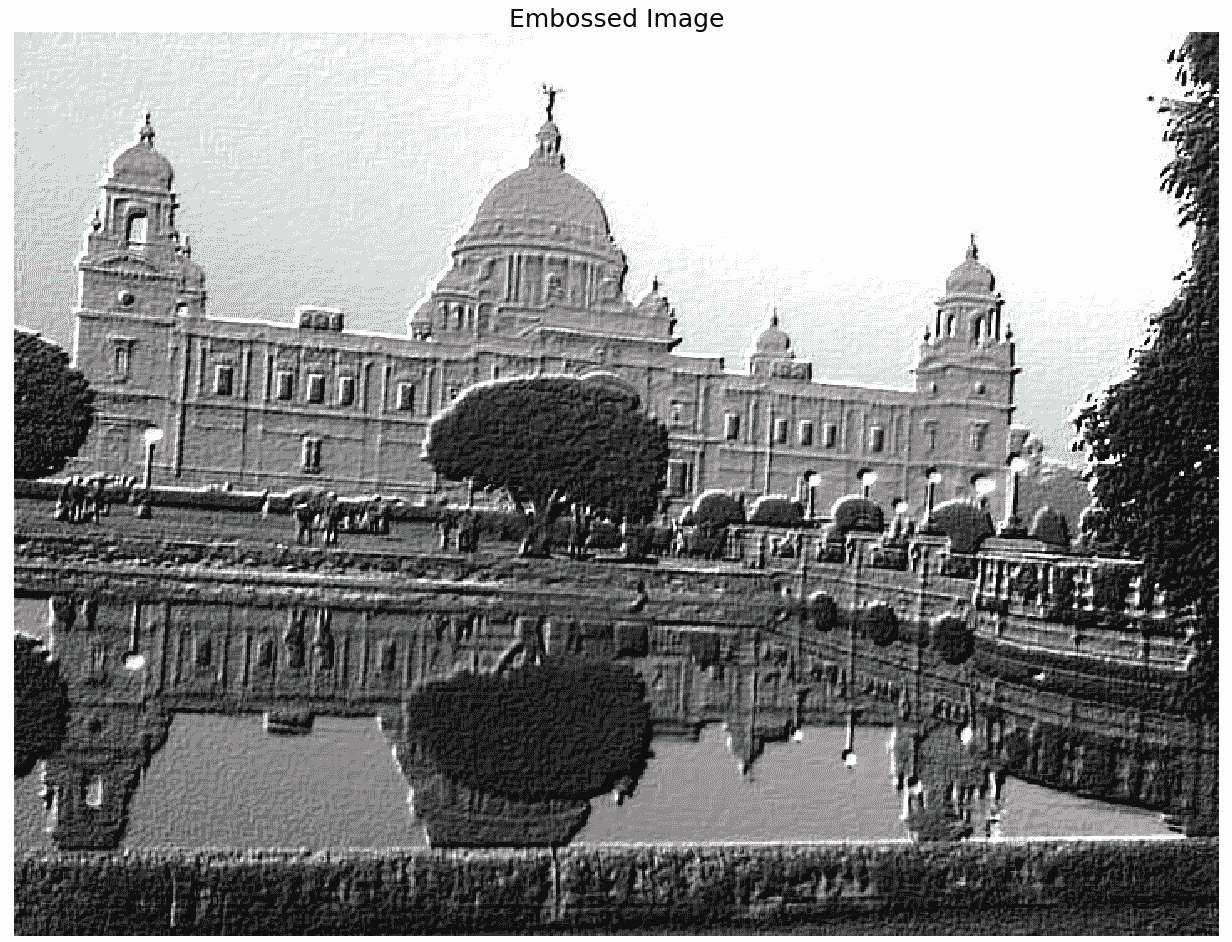# 相关与卷积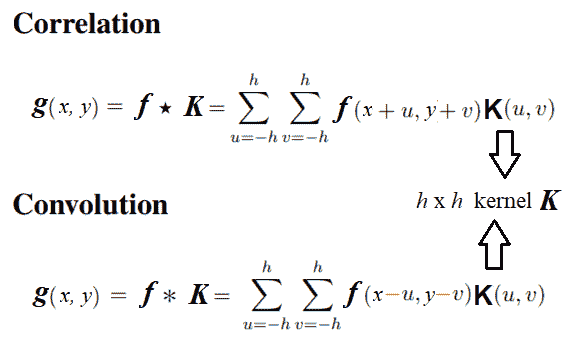SciPy 信号模块的correlated2d() ...

# 图像与模板互相关的模板匹配

face_image = misc.face(gray=True) - misc.face(gray=True).mean()
template_image = np.copy(face_image[300:365, 670:750]) # right eye
template_image -= template_image.mean()
face_image = face_image + np.random.randn(*face_image.shape) * 50 # add random noise
correlation = signal.correlate2d(face_image, template_image, boundary='symm', mode='same')
y, x = np.unravel_index(np.argmax(correlation), correlation.shape) # find the match
fig, (ax_original, ax_template, ax_correlation) = pylab.subplots(3, 1, figsize=(6, 15))
ax_original.imshow(face_image, cmap='gray')
ax_original.set_title('Original', size=20)
ax_original.set_axis_off()
ax_template.imshow(template_image, cmap='gray')
ax_template.set_title('Template', size=20)
ax_template.set_axis_off()
ax_correlation.imshow(correlation, cmap='afmhot')
ax_correlation.set_title('Cross-correlation', size=20)
ax_correlation.set_axis_off()
ax_original.plot(x, y, 'ro')
fig.show()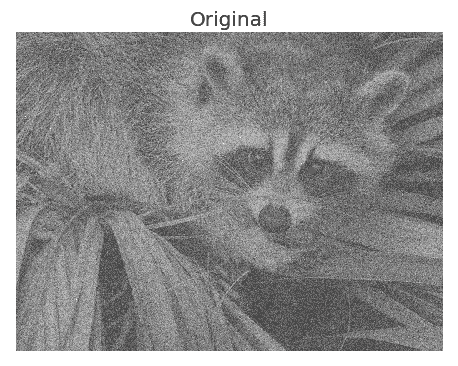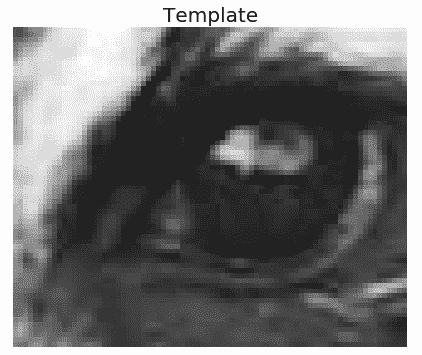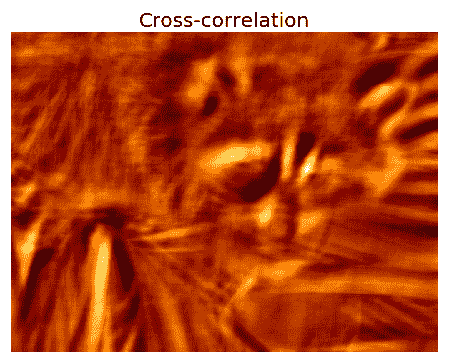# 问题

1. 使用高斯 LPF 实现下采样和消除混叠（提示：先应用高斯滤波器，然后每隔一行和一列进行滤波，将房屋灰度图像减少四次。在下采样之前，比较是否使用 LPF 进行预处理的输出图像）
2. 使用 FFT 对图像进行上采样：首先通过在每个交替位置填充零行/列，将lena灰度图像的大小增加一倍，然后使用 FFT，然后使用 LPF，然后使用 IFFT 获得输出图像。它为什么有效？
3. 尝试用彩色（RGB）图像应用傅里叶变换和图像重建。（提示：对每个通道分别应用 FFT）。
4. 用数学方法和 2D 核例子说明高斯核的傅里叶变换是另一个高斯核。

5. 使用lena图像和非对称 ripple 核生成具有相关和卷积的图像。显示输出图像不同。现在，翻转内核两次（颠倒和左右），并应用与翻转内核的相关性。输出图像是否与使用原始内核进行卷积获得的图像相同